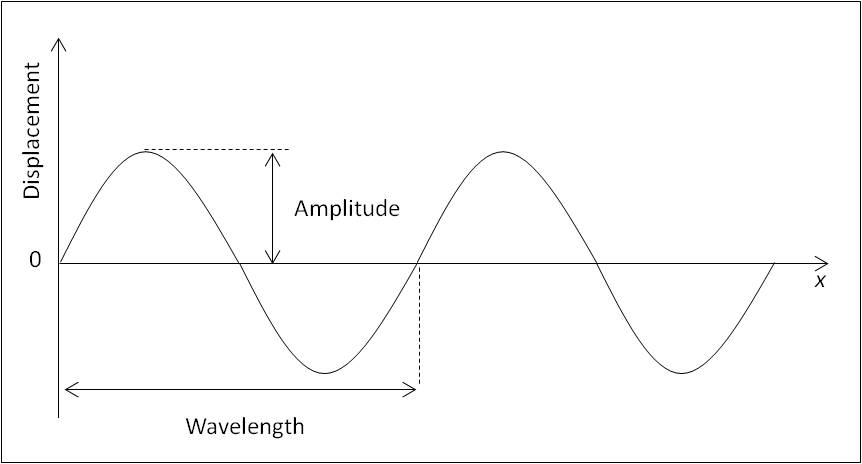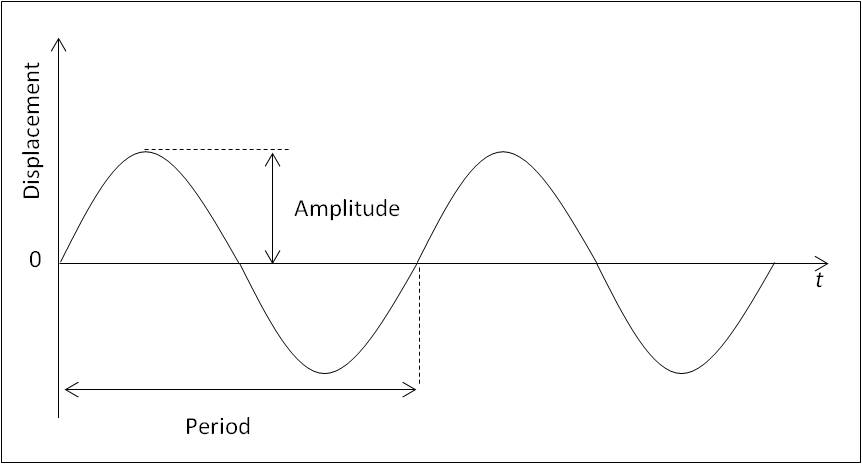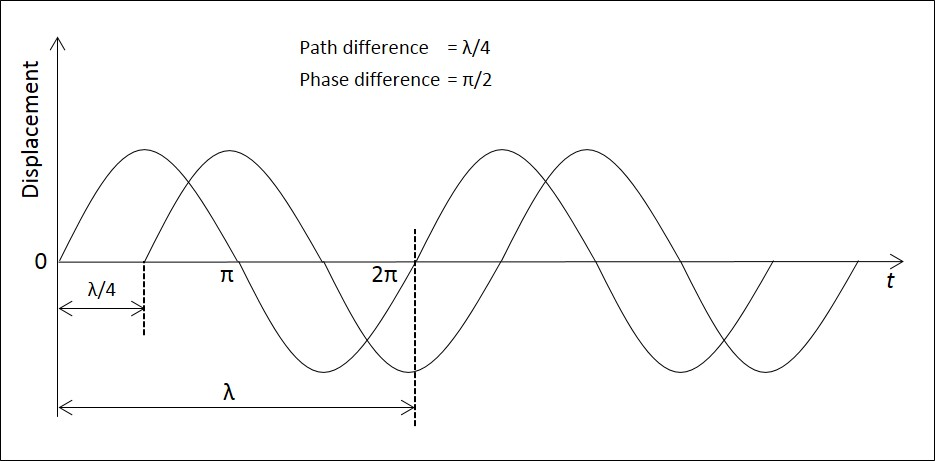# Progressive Waves

## Introduction

Waves, which move from place to place without the transfer of matter, are called progressive waves. In this article, important properties of progressive waves are explained.

A. Displacement

The displacement of a particle on a wave is its distance in a specified direction from its position.

A transverse wave is represented by plotting displacement on the y-axis and distance (x) along the wave in the direction of energy travel, on the x-axis. Even though, for a longitudinal wave, the displacement of the particles is along the direction of the energy travel, the displacement is plotted on the y-axis and distance along the x-axis. The graph for representing both transverse and longitudinal waves is the same in shape as represented by the figure below. Wave properties of waves can be determined by these graphs.Figure 1: Wave Properties

B. Amplitude

The amplitude of the wave motion is defined as the maximum displacement of a particle in the wave. Amplitude of a wave is shown in Figure 1.

C. Wavelength

It can be noted from Figure 1 that the wave repeats. The wave can be reconstructed by repeating a section of the wave. The length of the smallest repeated unit is called its wavelength.

The wavelength is the shortest distance between two peaks, or the shortest distance between two troughs. It can also be defined as the distance moved by the wavefront during one oscillation of the source of the waves. The symbol for wavelength is λ (Greek letter lambda).

D. Period

Waves can also be represented by plotting the displacement in the y-axis and time in the x-axis, as shown in Figure 2. The wave repeats after a certain interval of time. The time for one complete vibration is called the period (T) of the wave. The period of the wave is the time for a particle in the wave to complete one vibration, or one cycle.Figure 2: Period of a Wave

E. Frequency

The number of complete vibrations (cycles) per unit time is called the frequency (f) of the wave. For waves on ropes or strings, displacement and amplitude are measured in mm, m or other units of length. Period is measured in seconds (s). The unit for frequency is per second (s-1) or Hertz (Hz).

F. Phase

Phase is used to describe the relative positions of the crests or troughs of two different waves of the same frequency. When the crests and troughs of the two waves are aligned, the waves are said to be in phase. When crests and troughs are not aligned, the waves are said to have a phase difference. When a crest and a trough of two waves are aligned, the waves are said to be in antiphase. Phase is measured in degrees or radians.Figure 3: Phase Difference Between Two Waves

Consider the two waves shown in Figure 3. Both the waves are of the same frequency, but with a phase difference between them. The period (T) corresponds to a phase angle of 2π or 360°. The two waves are out of step by a time (t). Thus,Phase difference of the waves of wavelength λ, which are out of step by a distance x,From the definition of wavelength λ, in one cycle of the source, the wave energy moves a distance λ. The time taken for one cycle is the time period (T).G. Path difference

Difference in distance travelled by two waves is called the path difference. The phase difference may be produced by allowing the two sets of waves to travel different distances – this difference in distance of travel is called the path difference.

The two waves illustrated in the following figure have phase difference (π/2) and path difference(λ/4).Figure 4: Path Difference and Phase Difference

H. Summary

• Waves which move from place to place without the transfer of matter are called progressive waves.
• The displacement of a particle on a wave is its distance in a specified direction from its position.
• The amplitude of the wave motion is defined as the maximum displacement of a particle in the wave.
• The wavelength is the shortest distance between two peaks or the shortest distance between two troughs. It can also be defined as the distance moved by the wavefront during one oscillation of the source of the waves.
• The period of the wave is the time for a particle in the wave to complete one vibration, or one cycle.
• The number of complete vibrations (cycles) per unit time is called the frequency (f) of the wave.
• When the crests and troughs of the two waves are aligned, the waves are said to be in phase. When crests and troughs are not aligned, the waves are said to have a phase difference. When a crest and a trough of two waves are aligned, the waves are said to be in antiphase.
• Difference in distance travelled by two waves is called the path difference.